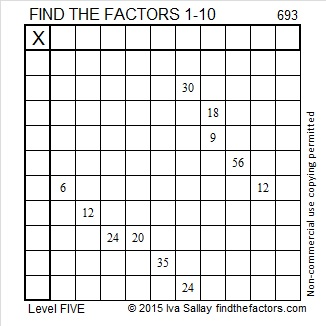# Divisibility Tricks Applied to 693

• 693 is a composite number.
• Prime factorization: 693 = 3 x 3 x 7 x 11, which can be written 693 = (3^2) x 7 x 11
• The exponents in the prime factorization are 2, 1, and 1. Adding one to each and multiplying we get (2 + 1)(1 + 1)(1 + 1) = 3 x 2 x 2 = 12. Therefore 693 has exactly 12 factors.
• Factors of 693: 1, 3, 7, 9, 11, 21, 33, 63, 77, 99, 231, 693
• Factor pairs: 693 = 1 x 693, 3 x 231, 7 x 99, 9 x 77, 11 x 63, or 21 x 33
• Taking the factor pair with the largest square number factor, we get √693 = (√9)(√77) = 3√77 ≈ 26.324893Some quick divisibility tricks applied to the number 693:

1. Every counting number is divisible by 1
2. 693 is not even so it isn’t divisible by 2
3. Every digit of 693 is divisible by 3, so 693 is divisible by 3
4. Since it isn’t divisible by 2, it isn’t divisible by 4
5. 693 doesn’t end in a 5 or 0, so it’s not divisible by 5
6. 693 is divisible by 3 but not by 2 so it isn’t divisible by 6
7. 69 – 2(3) = 63, a multiple of 7 so 693 is divisible by 7
8. Since it isn’t divisible by 2 or 4, it can’t be divisible by 8
9. 6 + 9 + 3 = 18, a multiple of 9 so 693 is divisible by 9
10. Since the last digit of 693 isn’t 0, it is not divisible by 10
11. 69 + 3 = 0, so 693 is divisible by 11

The divisibility tricks that worked on the number 693 are quite easy to see on the outside of this factor cake.Here is today’s factoring puzzle:Print the puzzles or type the solution on this excel file: 10 Factors 2015-11-23

———————————————————————————

693 is a palindrome in several bases:

• 1010110101 BASE 2; note 1(512) + 0(256) + 1(128) + 0(64) + 1(32) + 1(16) + 0(8) + 1(4) + 0(2) + 1(1) = 693
• 3113 BASE 6; note 3(216) + 1(36) + 1(6) + 3(1) = 693
• 414 BASE 13; note 4(169) + 1(13) + 4(1) = 693
• 313 BASE 15; note 3(225) + 1(15) + 3(1) = 693

———————————————————————————Ricardo tweeted his work for this puzzle, too.

//platform.twitter.com/widgets.js

This site uses Akismet to reduce spam. Learn how your comment data is processed.